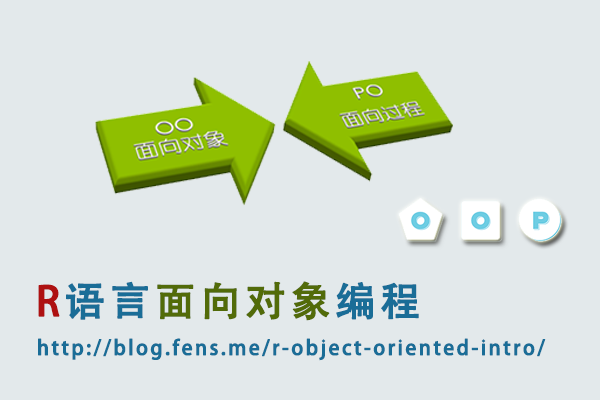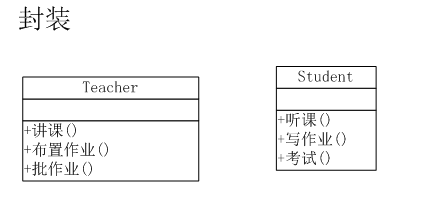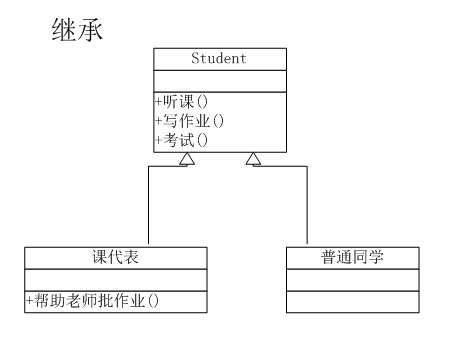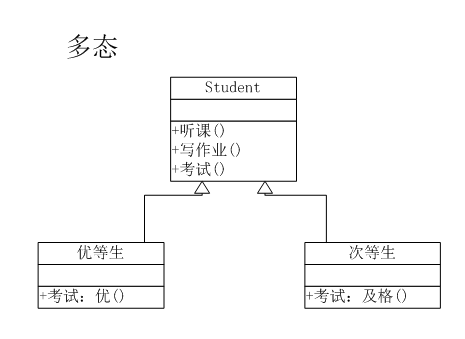## R语言面向对象编程

R的极客理想系列文章，涵盖了R的思想，使用，工具，创新等的一系列要点，以我个人的学习和体验去诠释R的强大。

R语言作为统计学一门语言，一直在小众领域闪耀着光芒。直到大数据的爆发，R语言变成了一门炙手可热的数据分析的利器。随着越来越多的工程背景的人的加入，R语言的社区在迅速扩大成长。现在已不仅仅是统计领域，教育，银行，电商，互联网….都在使用R语言。

• 张丹(Conan), 程序员Java,R,PHP,Javascript
• weibo：@Conan_Z
• blog: http://blog.fens.me
• email: bsspirit@gmail.com1. 什么是面向对象？
2. R为什么要进行面向对象编程?
3. R的面向对象编程
4. 与其他语言的对比

## 1 什么是面向对象？• 老师的行为：讲课，布置作业，批作业
• 学生的行为：听课，写作业，考试is a 和 has a

• is a: 为继承关系，比如 菱形、圆形和方形都是一种形状
• has a：为组合关系或聚合关系，比如 电脑是由显示器、CPU、硬盘等组成

## 2 R为什么要进行面向对象编程?

R主要面向统计计算，而且代码量一般不会很大，几十行，几百行，使用面向过程的编程方法就可以很好地完成编程的任务。

## 3 R的面向对象编程

R的面向对象编程是基于泛型函数(generic function)的，而不是基于类层次结构。接下来，我从面向对象的3个特征入手，分别用R语言进行实现，使用的案例为上文中，老师和学生的3幅图。

3.1 R语言实现封装

``````
# 定义老师对象和行为
> teacher <- function(x, ...) UseMethod("teacher")
> teacher.lecture <- function(x) print("讲课")
> teacher.assignment <- function(x) print("布置作业")
> teacher.correcting <- function(x) print("批改作业")
> teacher.default<-function(x) print("你不是teacher")

# 定义同学对象和行为
> student <- function(x, ...) UseMethod("student")
> student.attend <- function(x) print("听课")
> student.homework <- function(x) print("写作业")
> student.exam <- function(x) print("考试")
> student.default<-function(x) print("你不是student")

# 定义两个变量，a老师和b同学
> a<-'teacher'
> b<-'student'

# 给老师变量设置行为
> attr(a,'class') <- 'lecture'
# 执行老师的行为
> teacher(a)
 "讲课"

# 给同学变量设置行为
> attr(b,'class') <- 'attend'
# 执行同学的行为
> student(b)
 "听课"

> attr(a,'class') <- 'assignment'
> teacher(a)
 "布置作业"

> attr(b,'class') <- 'homework'
> student(b)
 "写作业"

> attr(a,'class') <- 'correcting'
> teacher(a)
 "批改作业"

> attr(b,'class') <- 'exam'
> student(b)
 "考试"

# 定义一个变量，既是老师又是同学
> ab<-'student_teacher'
# 分别设置不同对象的行为
> attr(ab,'class') <- c('lecture','homework')
# 执行老师的行为
> teacher(ab)
 "讲课"
# 执行同学的行为
> student(ab)
 "写作业"
``````

3.2 R语言实现继承

``````
# 给同学对象增加新的行为
> student.correcting <- function(x) print("帮助老师批改作业")

# 辅助变量用于设置初始值
> char0 = character(0)

# 实现继承关系
> create <- function(classes=char0, parents=char0) {
+     mro <- c(classes)
+     for (name in parents) {
+         mro <- c(mro, name)
+         ancestors <- attr(get(name),'type')
+         mro <- c(mro, ancestors[ancestors != name])
+     }
+     return(mro)
+ }

# 定义构造函数，创建对象
> NewInstance <- function(value=0, classes=char0, parents=char0) {
+     obj <- value
+     attr(obj,'type') <- create(classes, parents)
+     attr(obj,'class') <- c('homework','correcting','exam')
+     return(obj)
+ }

# 创建父对象实例
> StudentObj <- NewInstance()

# 创建子对象实例
> s1 <- NewInstance('普通同学',classes='normal', parents='StudentObj')

# 给课代表，增加批改作业的行为
> attr(s2,'class') <- c(attr(s2,'class'),'correcting')

# 查看普通同学的对象实例
> s1
 "普通同学"
attr(,"type")
 "normal"     "StudentObj"
attr(,"class")
 "homework"   "attend" "exam"

# 查看课代表的对象实例
> s2
 "课代表"
attr(,"type")
attr(,"class")
 "homework"   "attend" "exam"       "correcting"
``````

3.3 R语言实现多态

``````
# 创建优等生和次等生，两个实例
> e1 <- NewInstance('优等生',classes='excellent', parents='StudentObj')
> e2 <- NewInstance('次等生',classes='poor', parents='StudentObj')

# 修改同学考试的行为，大于85分结果为优秀，小于70分结果为及格
> student.exam <- function(x,score) {
+     p<-"考试"
+     if(score>85) print(paste(p,"优秀",sep=""))
+     if(score<70) print(paste(p,"及格",sep=""))
+ }

# 执行优等生的考试行为，并输入分数为90
> attr(e1,'class') <- 'exam'
> student(e1,90)
 "考试优秀"

# 执行次等生的考试行为，并输入分数为66
> attr(e2,'class') <- 'exam'
> student(e2,66)
 "考试及格"
``````

## 4 R的面向过程编程

4.1 定义老师和同学两个对象和行为

``````
# 辅助变量用于设置初始值
> char0 = character(1)

# 定义老师对象和行为
> teacher_fun<-function(x=char0){
+     if(x=='lecture'){
+         print("讲课")
+     }else if(x=='assignment'){
+         print("布置作业")
+     }else if(x=='correcting'){
+         print("批改作业")
+     }else{
+         print("你不是teacher")
+     }
+ }

# 定义同学对象和行为
> student_fun<-function(x=char0){
+     if(x=='attend'){
+         print("听课")
+     }else if(x=='homework'){
+         print("写作业")
+     }else if(x=='exam'){
+         print("考试")
+     }else{
+         print("你不是student")
+     }
+ }

# 执行老师的一个行为
> teacher_fun('lecture')
 "讲课"

# 执行同学的一个行为
> student_fun('attend')
 "听课"
``````

4.2 区别普通同学和课代表的行为

``````
# 重定义同学的函数，增加角色判断
> student_fun<-function(x=char0,role=0){
+     if(x=='attend'){
+         print("听课")
+     }else if(x=='homework'){
+         print("写作业")
+     }else if(x=='exam'){
+         print("考试")
+     }else if(x=='correcting'){
+         if(role==1){#课代表
+             print("帮助老师批改作业")
+         }else{
+             print("你不是课代表")
+         }
+     }else{
+         print("你不是student")
+     }
+ }

# 以普通同学的角色，执行课代表的行为
> student_fun('correcting')
 "你不是课代表"

# 以课代表的角色，执行课代表的行为
> student_fun('correcting',1)
 "帮助老师批改作业"
``````

4.3 参加考试，以成绩区别出优等生和次等生

``````
# 修改同学的函数定义，增加考试成绩参数
> student_fun<-function(x=char0,role=0,score){
+     if(x=='attend'){
+         print("听课")
+     }else if(x=='homework'){
+         print("写作业")
+     }else if(x=='exam'){
+         p<-"考试"
+         if(score>85) print(paste(p,"优秀",sep=""))
+         if(score<70) print(paste(p,"及格",sep=""))
+     }else if(x=='correcting'){
+         if(role==1){#课代表
+             print("帮助老师批改作业")
+         }else{
+             print("你不是课代表")
+         }
+     }else{
+         print("你不是student")
+     }
+ }

# 执行考试函数，考试成绩为大于85分，为优等生
> student_fun('exam',score=90)
 "考试优秀"

# 执行考试函数，考试成绩为小于70分，为次等生
> student_fun('exam',score=66)
 "考试及格"
``````This entry was posted in R语言实践

Article Rating
Subscribe
Notify ofThis site uses Akismet to reduce spam. Learn how your comment data is processed.

Inline Feedbacks(另外，如果能把代码块中每行前面的 > 和 + 去掉，就太好了。)

lecture <- function(x, …) UseMethod("lecture")

assignment <- function(x, …) UseMethod("assignment")

exam <- function(x, …) UseMethod("exam")

lecture.teacher <- function(x) print("讲课")

assignment.teacher <- function(x) print("布置作业")

exam.teacher <- function(x) print("出考卷")

lecture.student <- function(x) print("听课")

assignment.student <- function(x) print("写作业")

exam.student <- function(x) print("考试")

a<-'teacher'

b<-'student'

class(a) <- 'teacher'

class(b) <- 'student'

lecture(a)

lecture(b)

assignment(a)

assignment(b)

exam(a)

exam(b)1. generic function是S3对象的实现，还有S4和RC另外两种对象实现，后面会写。
2. 面向对象设计，主要是为了解决代码结构设计问题，文章中已经写了。

3. <和+，你可复制出来，自己替换一下就行了。print.student <- function(x){}

a <- structure("a", class = "student")
print(a)

attr(a,'class') <- 'print'
student(a)S3只是一种通过generic function的面向对象的模拟实现，从根本上并不适合于完全面向对象的设计。所以，才会出现你的这样的设计；同时有一些R的内置S3对象，也是你这样的设计。回到R语言本身，R的自由性，就是这种不够标准化的设计。

S4和RC，会比S3有更严格的结构性要求，会避免上面的问题。[…] 关于面向对象的介绍，请参考文章：R语言面向对象编程 […]> student(s1)
 “写作业”
> student(s2)
 “写作业”http://blog.fens.me/r-class-s3/🙂🙂mro <- c(mro, ancestors[ancestors != name])create <- function(classes=char0, parents=char0) {
mro <- c(classes)
for (name in parents) {
mro <- c(mro, name)
ancestors <- attr(get(name),'type')
mro <- c(mro, ancestors[ancestors != name])
}
return(mro)
}s3类似的class属性，是以字符串定义的。就是把所的父类继承给子类

18
0